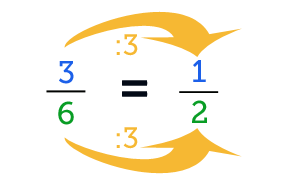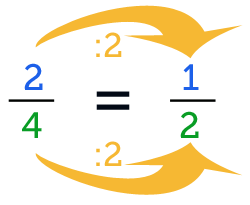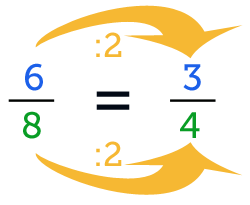# Simplifying Fractions

## What is simplifying a fractionFor every fraction there is a simplest form. The simplest form is a fraction in which the numerator (the top number) and the denominator (the bottom number) are as small as possible. Simplifying fractions means making the fraction as small as possible.
An example of simplifying a fraction: 36 12

Are the terms described here difficult? Have a look at the 'What Is a Fraction?' Page for more explanation first.

On this page you will find examples and exercices. For an extensive practice go to one of the 5-step plans.

## How do you simplify a fraction?

To simplify a fraction you look for the greatest common factor (GCF). By greatest common factor we mean the highest number you can divide both the numerator and the denominator by. You then need to divide the numerator and the denominator by that number to make the fraction as simple as possible.
Below are examples with explanation and exercises

### Example 1

We're going to simplify the fraction 24.

24 numeratordenominator

What is the greatest common factor of 24?
The numerator is 2 and you can only divide it by 1 and 2.
The denominator is 4 and you can divide it by 1, 2, and 4.

Then you have to look for the highest number you can use to divide both. That's 2, so the greatest common factor is 2.
2(numerator) divided by 2 is 1. 4(denominator) divided by 2 is 2. This gives you the simplified fraction 12.### Exercise 1:

Number of questions:

Time per question:

Simplify the fraction, the denominators are already entered. Choose the number of sums and the time per sum and click start.

Tip: use tab to go to the next field

### Exercise 2:

Number of questions:

Time per question:

Simplify the fraction. Choose the number of sums and the time per sum and click start.

Tip: use tab to go to the next field

### Example 2

We're going to simplify the fraction 68. ​

First we'll look for the greatest common denominator.
The numerator is 6, this can be divided by 2,3, and 6.
The denominator is 8, this can be divided by 2,4, and 8.
The greatest common factor for 68 is 2. This makes the simplified fraction 34.### Example 3

We're going to simplify the fraction 1624.

16(numerator) can be divided by: 2, 4, 8, and 16.
24(denominator) can be divided by: 2,3, 4, 6, 8, 12, and 24.
The greatest common factor for 1624 is 8. 16 divided by 8 is 2 and 24 divided by 8 is 3.
This makes the simplified fraction 23.